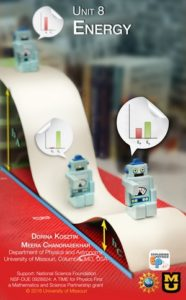### Unit 8 Energy      View Unit 8Building on knowledge from previous units, students connect forces and motion to energy. Students design their own experiments to study different types of energy storage, energy transfers and transformations. By the end of the unit, students will be able to answer the following question:

• What are the different types of energy storage?
• How can one represent energy transfers and transformations using pie charts and bar graphs?
• How is energy related to work?
• How can we calculate the energy stored in a system?
• How do you mathematically calculate the kinetic energy, elastic and gravitational potential energy?
• How can you use the conservation of energy theorem to find position or velocity of an object?

Suggested timeline for teaching Unit 8

Big Ideas
1. Energy is related to work that is done by a force.
2. Energy can be stored, transferred or transformed.
3. For a closed system, energy is conserved.

Learning Goals and Objectives
By the end of this unit, the students should be able to:

1. Differentiate between energy transfer, transformation and storage. (DOK3) a) Define open or closed physical systems for a given situation involving energy. b) Identify and compare different forms of energy storage: gravitational potential energy, elastic potential energy, kinetic energy, thermal energy. c) Identify the initial and final states of a physical system for a given energy transfer and/or transformation process. d) Recognize that energy is conserved in a closed system.
2. Analyze a physical system in terms of energy storage, transfer and transformation using various representational tools (pictorial and/or verbal descriptions, pie charts, and bar graphs). (DOK4) a) Distinguish between isolated and non-isolated systems. b) Operationally define an isolated system such that no energy transfers are occurring into or out of the system. c) Identify the forms of energy storage for a system in a given state. d) Use pie charts to represent the energy transfer and transformation when a system goes from one state to another. e) Use bar graphs to represent the energy transfer and transformation when a system goes from one state to another. f) Relate energy transfers to external interactions.
3. Relate the concept of work to an energy transfer mechanism. (DOK2) a) Demonstrate that a system has the potential to do work. b) Give examples of the three methods of energy transfer, e.g., working, heating and radiation. c) Relate work to the external net force applied on the system and its displacement. d) Relate work to the transfer of energy into or out of the system.
4. Design and conduct experiments to determine the energy stored in a system. (DOK4) a) Use technology (i.e., force probes) to measure and collect data to determine energy stored in a given system. b) Conduct an experiment to determine the relationship between gravitational potential energy and vertical height of an object with respect to a chosen reference frame. c) Relate the change in the gravitational potential energy of a system to the work done by the gravitational force. d) Conduct an experiment to determine the relationship between the elastic potential energy stored in a spring and its deformation. e) Relate the change in the elastic potential energy of a system to the work done by the elastic force. f) Conduct an experiment to determine the relationship between the kinetic energy stored in a moving object and the speed of the object. g) Conduct an experiment to determine the power output of a person. h) Relate the power to the rate of change of energy with time.
5. Mathematically and graphically determine work, gravitational potential energy, elastic potential energy, kinetic energy, and power. (DOK3) a) Determine a mathematical expression for calculating work when the force and displacement of an object along the direction of the force is known. b) Determine the units used for measuring work and thus energy. c) Recognize that work is a physical quantity that can have both positive and negative values. d) Calculate work done by a force as the i) Product of that force and the displacement of the object along the direction of that force, ii) Area under the force vs. displacement graph. e) Relate work and power to energy. f) Use the mathematical relationships for calculating energy with their corresponding units in problem-solving. g) Apply conservation of energy theorem in solving problems.